# Class 11 Maths NCERT Solutions for Chapter 3 Trigonometric Functions Exercise 3.4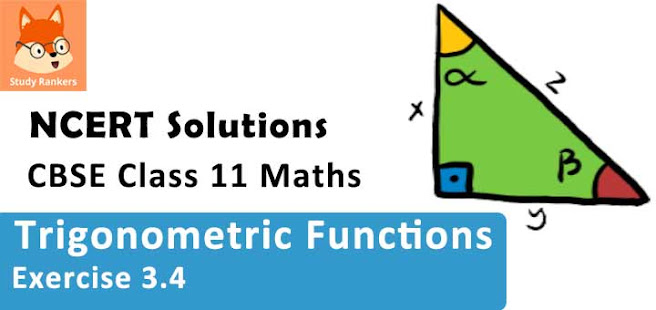### Trigonometric Functions Exercise 3.4 Solutions

1. Find the principal and general solutions of the equation tan x = √x

Solution

tan x = √x
It is known that tan(π/3) = √3 and tan (4π/3) = tan(π + π/3) = tan (π/3) = √3
Therefore, the principal solutions are x = π/3 and 4π/3.
Now, tan x = tan (π/3)
⇒ x = nπ + π/3, where n ∈ Z
Therefore, the general solution is x = nπ + π/3, where n ∈ Z

2. Find the principal and general solutions of the equation sec x = 2

Solution

sec x = 2
It is known that sec(π/3) = 2 and sec(5π/3) = sec(2π - π/3) = sec(π/3) = 2
Therefore, the principal solutions are x = π/3 and 5π/3.
Now, sec π = sec(π/3)
⇒ cos x = cos (π/3)  [sec x = 1/cos x]
⇒ x = 2nπ ± π/3, where n ∈ Z
Therefore, the general solution is x = 2nπ ± π/3, where n ∈ Z

3. Find the principal and general solutions of the equation cot x = -√3

Solution

cos x = -√3
It is known that cot (π/6) = √3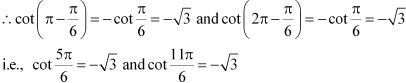Therefore, the principal solutions are x = 5π/6 and  11π/6.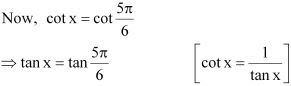⇒ x = nπ + 5π/6, where n ∈ Z
Therefore, the general solution is x = nπ + 5π/6, where n ∈ Z

4. Find the general solution of cosec x = –2

Solution

cosec x = -2
It is known that
cosec (π/6) = 2
∴ cosec(π + π/2) = -cosec(π/2) = -2 and cosec(2π - π/6) = -cosec (π/6) = -2
i.e., cosec (7π/6) = -2 and cosec(11x/6) = -2
Therefore, the principal solution are x = 7π/6 and 11π/6.
Now, cosec x = cosec(7π/6)
⇒ sin x = sin(7π/6)  [cosec x = 1/sin x]
⇒ x = nπ + (-1)n (7π/6) , where n ∈ Z
Therefore, the general solution is x = nπ + (-1)n (7π/6) where n ∈ Z.

5. Find the general solution of the equation cos 4x = cos 2x

Solution

cos 4x = cos 2x
⇒ cos 4x - cos 2x = 0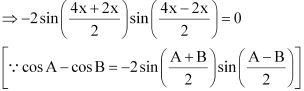⇒ sin 3x sin x = 0
⇒ sin 3x = 0 or sin x = 0
∴ 3x = nπ or x = nπ, where n ∈ Z
⇒ x = nπ/3  or x = nπ, where n ∈ Z

6. Find the general solution of the equation cos 3x + cos x – cos 2x = 0

Solution

cos 3x  + cos x - cos 2x = 0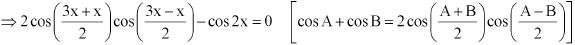⇒ 2 cos 2x cos x - cos 2x = 0
⇒ cos 2x (2 cos x - 1) = 0
⇒ cos 2x = 0 or 2 cos x - 1 = 0
⇒ cos 2x = 0 or cos x = 1/2
∴ 2x = (2n + 1)π/2 or cos x = cos π/3, where n ∈ Z
⇒ x = (2n + 1)π/4 or x = 2nπ ± π/3, where n ∈ Z

7. Find the general solution of the equation sin 2x + cos x = 0

Solution

sin 2x + cos x = 0
⇒ 2 sin x cos x + cos x = 0
⇒ cos x = 0 or  2 sin x + 1 = 0
Now, cos x = 0
⇒ cos x = (2n+1)π/2, where n ∈ Z
2 sin x + 1 = 0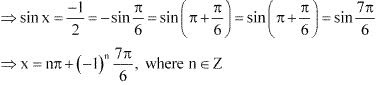Therefore, the general solution is (2n + 1)π/2 or nπ + (-1)n 7π/6, n ∈ Z.

8. Find the general solution for each of the following equations sec2 2x = 1– tan 2x

Solution

sec2 2x = 1– tan 2x
⇒ 1 + tan2 2x = 1 - tan 2x
⇒ tan2 2x + tan 2x = 0
⇒ tan 2x(tan 2x + 1) = 0
⇒ tan 2x = 0   or tan 2x + 1 = 0
Now, tan 2x = 0
⇒ tan 2x = tan 0
⇒ 2x = nπ + 0 , where n ∈ Z
⇒ x = nπ/2 , where n ∈ Z
tan 2x + 1 = 0
⇒ tan 2x = -1 = -tan(π/4) = tan(π - π/4) = tan(3π/4)
⇒ 2x = nπ + 3π/4, where n ∈ Z
⇒ x = nπ/2 + 3π/8, where n ∈ Z
Therefore, the general solution is nπ/2 or nπ/2 + 3π/8 , n ∈ Z.

9. Find the general solution of the equation sin x + sin 3x + sin 5x = 0

Solution

sin x + sin 3x + sin 5x = 0
(sin x + sin 5x) + sin 3x = 0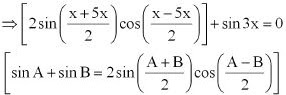⇒ 2 sin 3x cos(-2x) + sin 3x  = 0
⇒ 2 sin 3x cos 2x + sin 3x = 0
⇒ sin 3x(2 cos 2x + 1) = 0
⇒ sin 3x = 0 or 2 cos 2x + 1 = 0
Now, sin 3x = 0 ⇒ 3x = nπ , where n ∈ Z
i.e., x = nπ/3, where n ∈ Z
i.e., x = nπ/3, where n ∈ Z
2 cos 2x + 1 = 0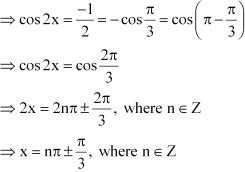Therefore, the general solution is nπ/3 or nπ ± π/3, n ∈ Z.